# Dirichlet's theorem on primes in arithmetic progressions

## Statement

Letbe relatively prime natural numbers. Then, there exist infinitely many primessuch that:.

For fixed, the primes that are congruent tomoduloare termed Dirichlet primes.

## Related facts

### Conjectures/facts about the first Dirichlet prime

• Heath-Brown's conjecture on the first Dirichlet prime: A conjecture, saying that the first Dirichlet prime in a given congruence class modulois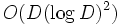.
• Chowla's conjecture on the first Dirichlet prime: A conjecture, saying that the first Dirichlet prime in a given congruence class modulois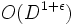.
• Chowla's corollary to generalized Riemannn hypothesis: Proved conditional to the generalized Riemann hypothesis, saying that the first Dirichlet prime in a given congruence class modulois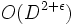.
• Linnik's theorem: An unconditional theorem, saying that there exists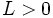such that the first Dirichlet prime in a given congruence class modulois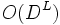. Heath-Brown showed that we can take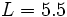.

### Conjectures/facts about contiguous blocks of Dirichlet primes

• Green-Tao theorem: This states that for any, there exists a prime arithmetic progression of length: an arithmetic progression of length, all of whose members are primes.

### Conjectures that generalize to higher degree polynomials

• Bunyakovsky conjecture is a conjecture for polynomials of degree two or more whose analogue for linear polynomials would be Dirichlet's theorem.